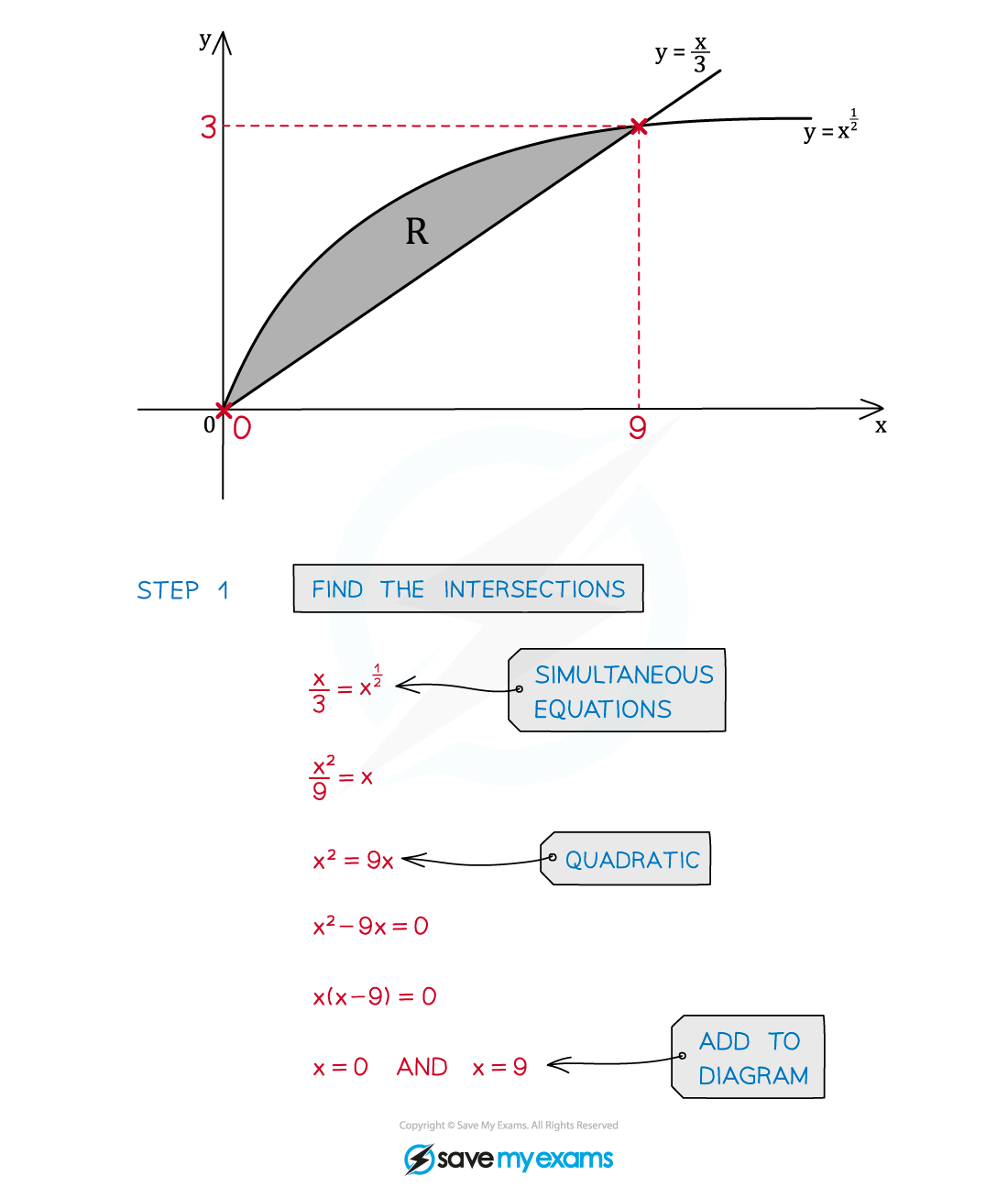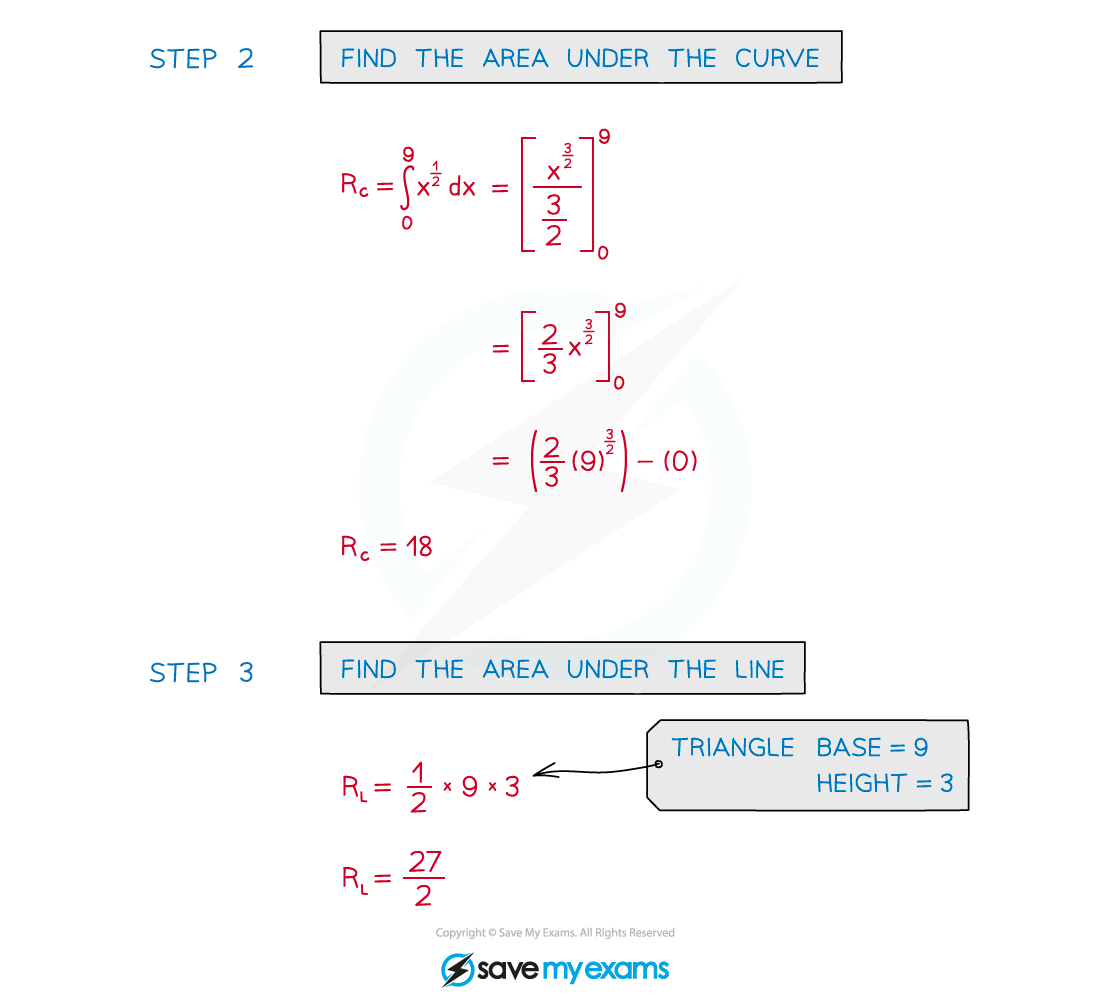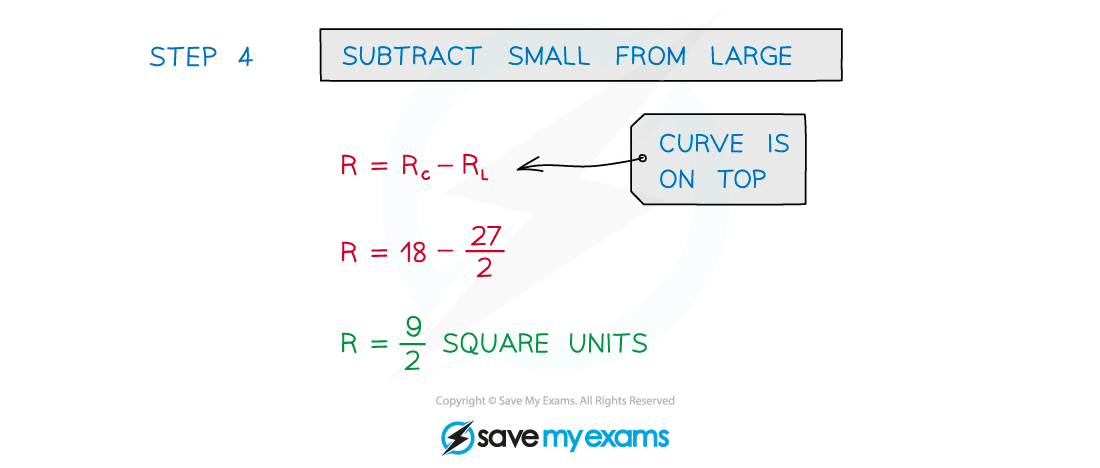# AQA A Level Maths: Pure复习笔记8.1.5 Area between a curve and a line

### Area between a curve and a line

#### What is the area between a curve and a straight line?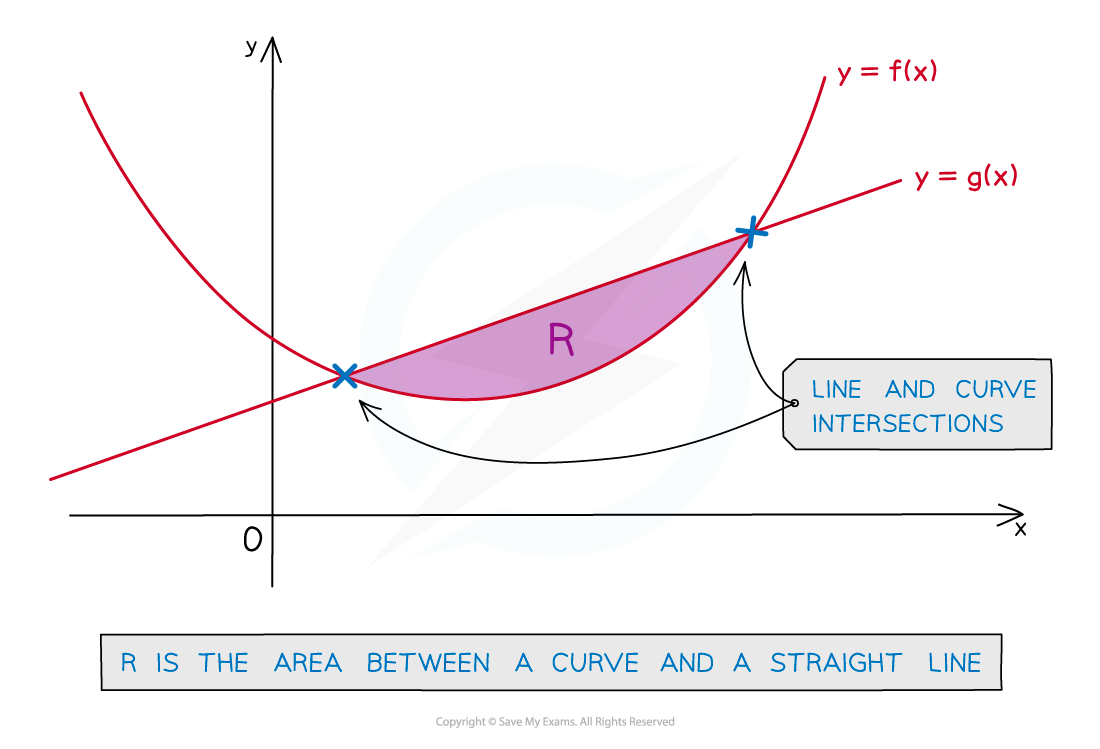• This is the area enclosed by a curve and a line between their intersections
• The intersections may need to be found using simultaneous equations

#### How do I find the area between a curve and a line?

• The area enclosed will be the difference between …
• the area under the curve and
• the area under the line
• These can be found separately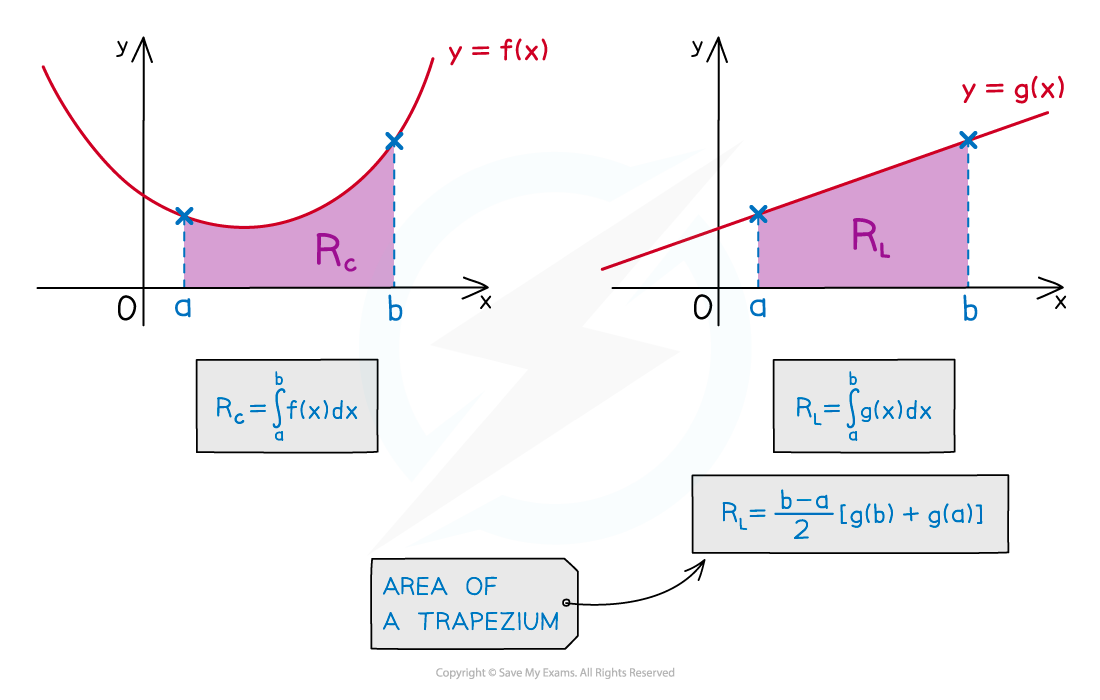• There may be easier ways to find the area under a line• Once the areas are found a question can be answered in full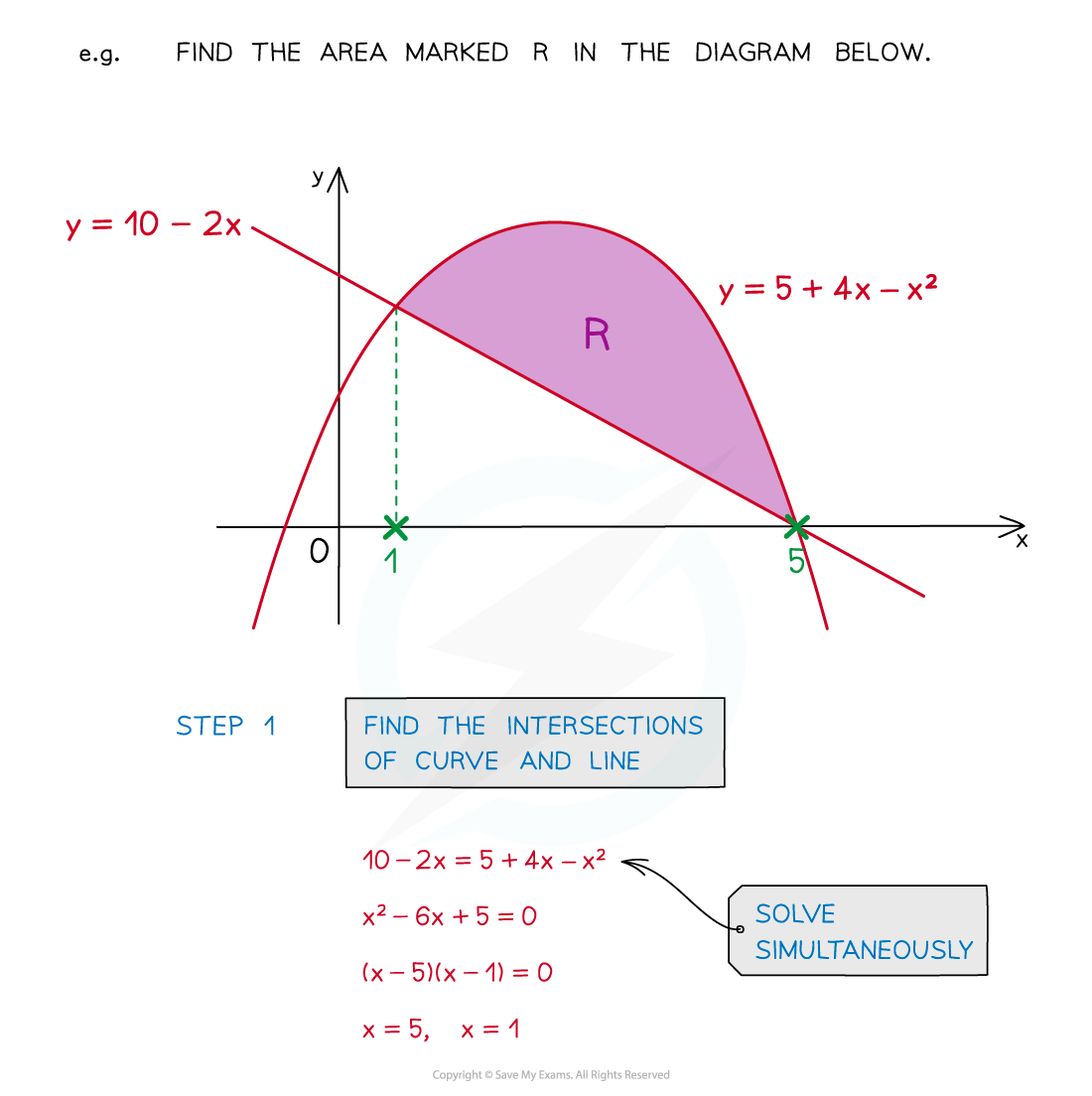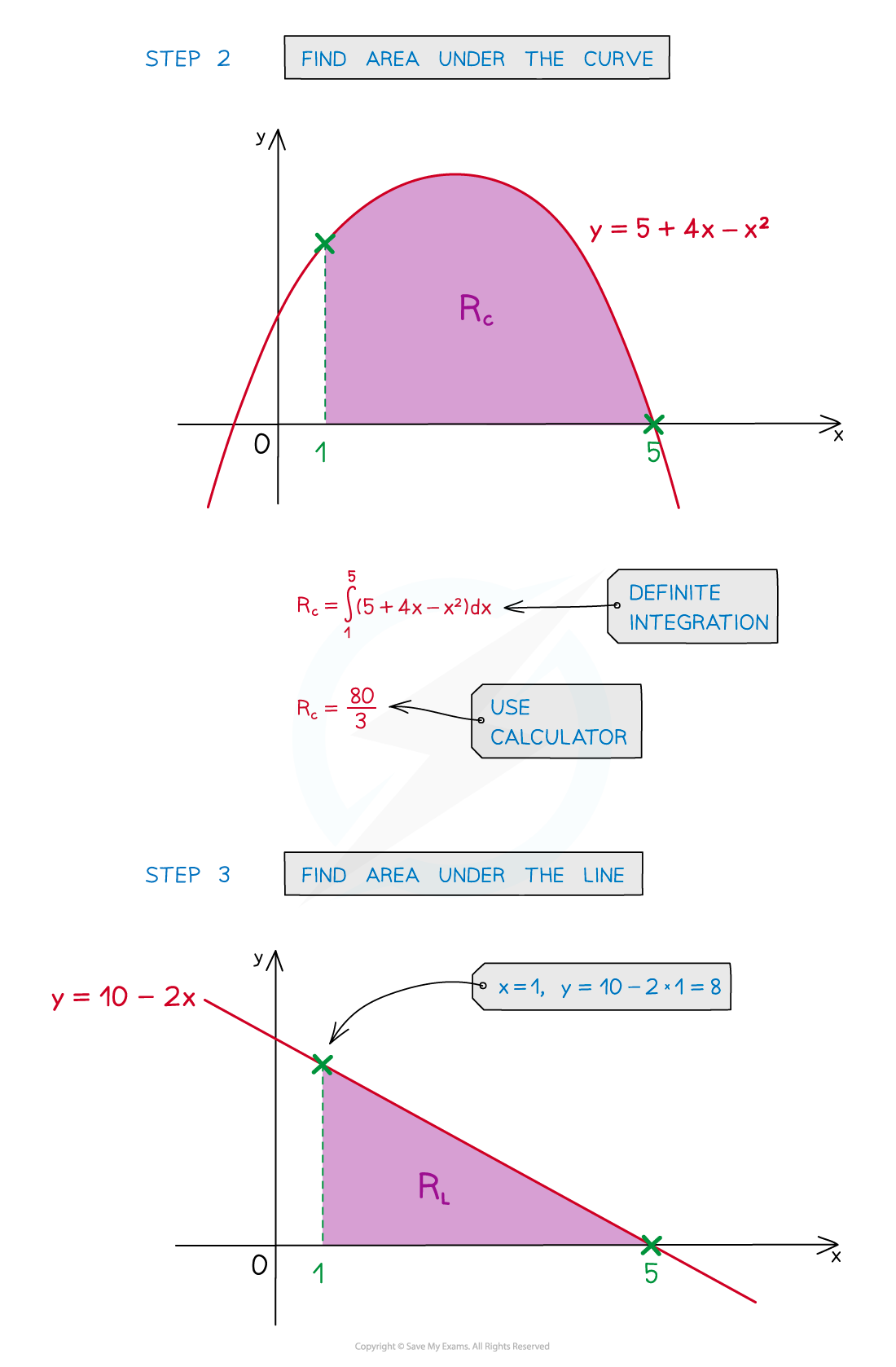• STEP 1: Find the intersections of the line and the curve
• STEP 2: Find the area under a curve, RC, using definite integration
• STEP 3: Find the area under a line, RL, either using definite integration or the area formulae for basic shapes
• STEP 4: To find the area, R, between the curve and the line subtract the smaller area from the larger area
• If curve on top this will be R– RL
• If line on top this will be RL - RC

#### Alternative method

• An alternative method allows subtraction of the two functions first
• But be extremely careful as to whether the curve or the line is on top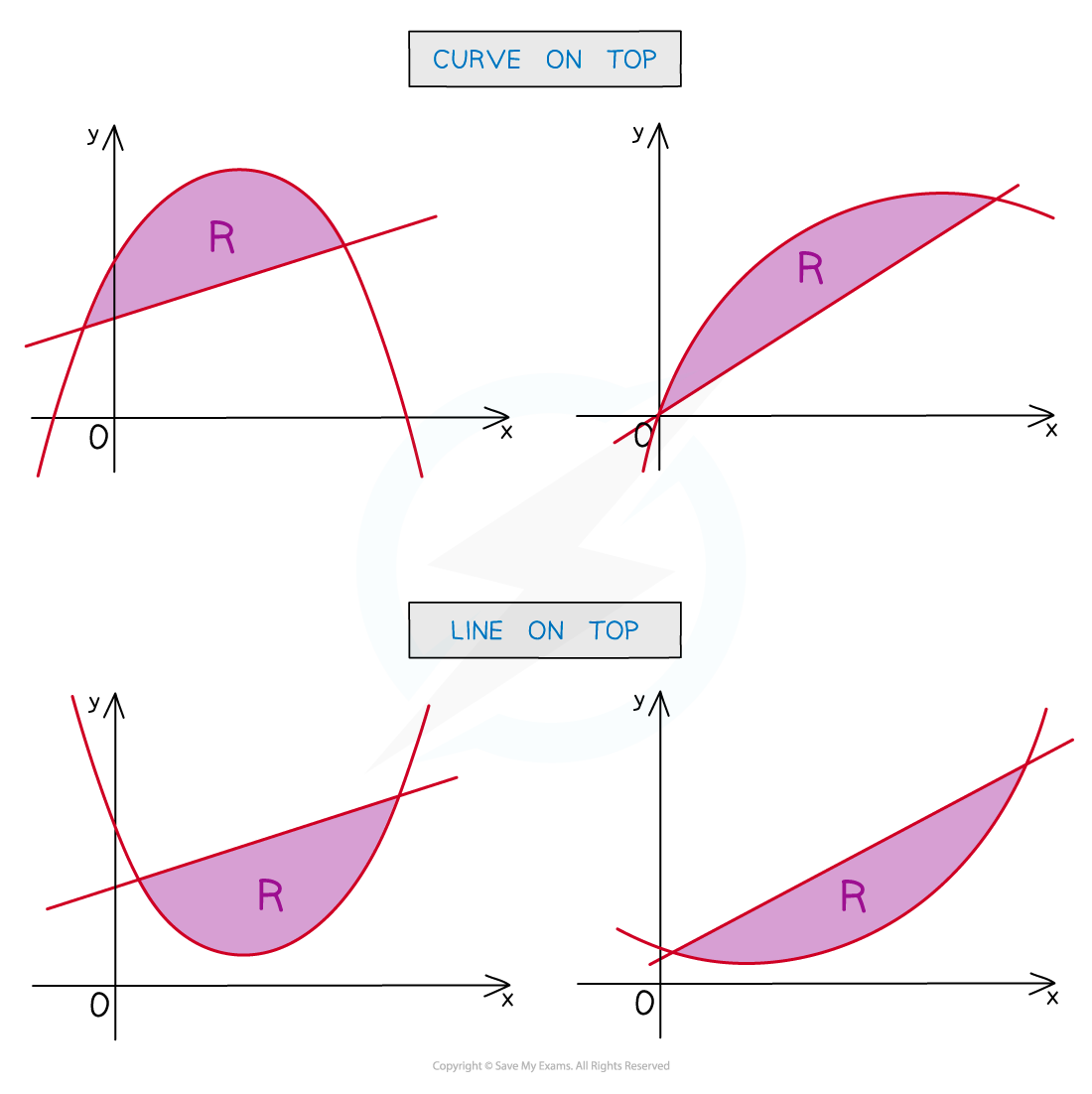• STEP 1: Find the intersections of the line and the curve
• STEP 2: Subtract the functions algebraically
• Ensure this is done the correct way round!
• STEP 3: Find the area, R, between the curve and the line using definite integration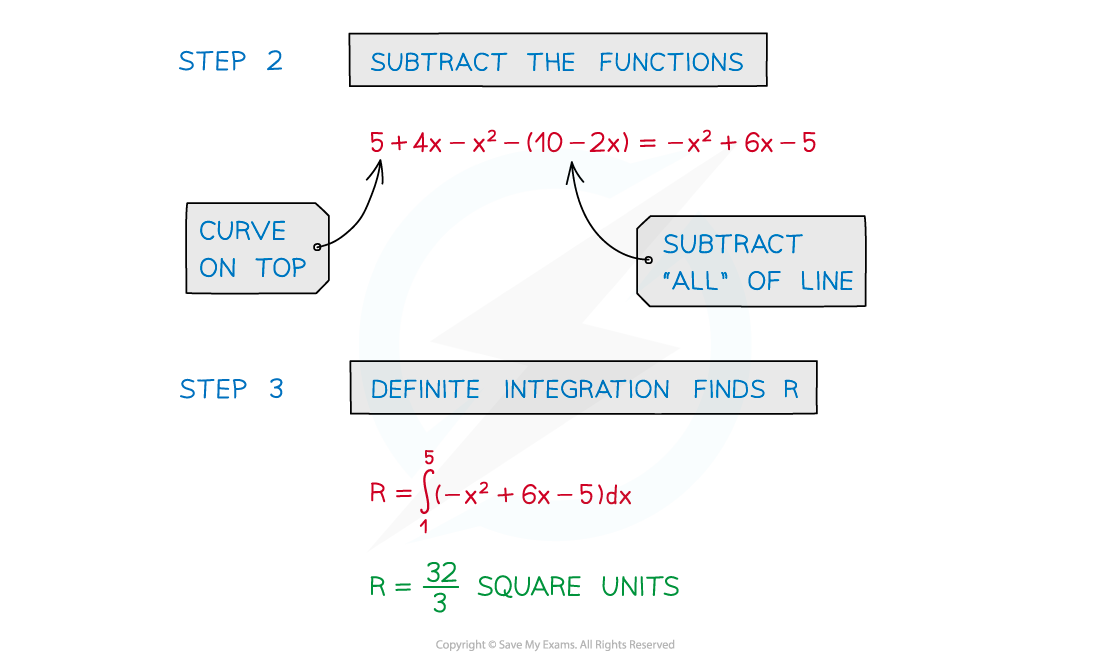#### Exam Tip

• Add information to any diagram provided
• Add axes intercepts, as well as intercepts between lines and curves
• Mark and shade the area you’re trying to find
• If no diagram provided, sketch one!

#### Worked Example